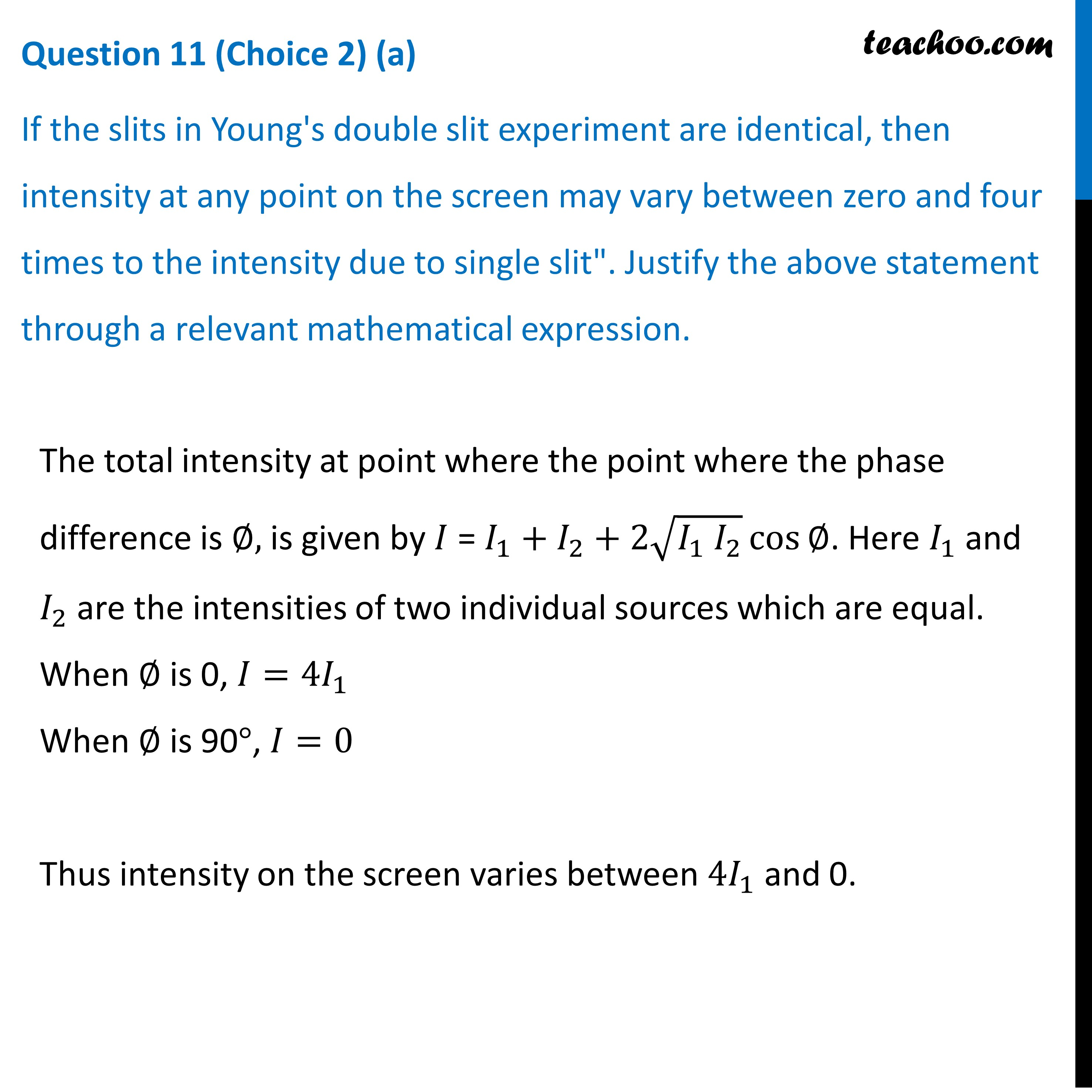CBSE Class 12 Sample Paper for 2022 Boards [Term 2] - Physics

Physics Class 12
Solutions to CBSE Sample Paper - Physics Class 12

## (a) "If the slits in Young's double slit experiment are identical, then intensity at any point on the screen may vary between zero and four times to the intensity due to single slit". Justify the above statement through a relevant mathematical expression.Learn in your speed, with individual attention - Teachoo Maths 1-on-1 Class

### Transcript

Question 11 (Choice 2) (a) If the slits in Young's double slit experiment are identical, then intensity at any point on the screen may vary between zero and four times to the intensity due to single slit". Justify the above statement through a relevant mathematical expression. The total intensity at point where the point where the phase difference is ∅, is given by 𝐼 = 𝐼_1+𝐼_2+2√(𝐼_1 𝐼_2 ) cos⁡"∅" . Here 𝐼_1 and 𝐼_2 are the intensities of two individual sources which are equal. When ∅ is 0, 𝐼=〖4𝐼〗_1 When ∅ is 90°, 𝐼=0 Thus intensity on the screen varies between 〖4𝐼〗_1 and 0.